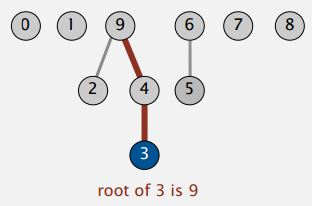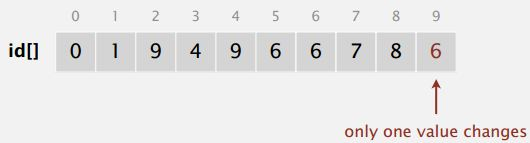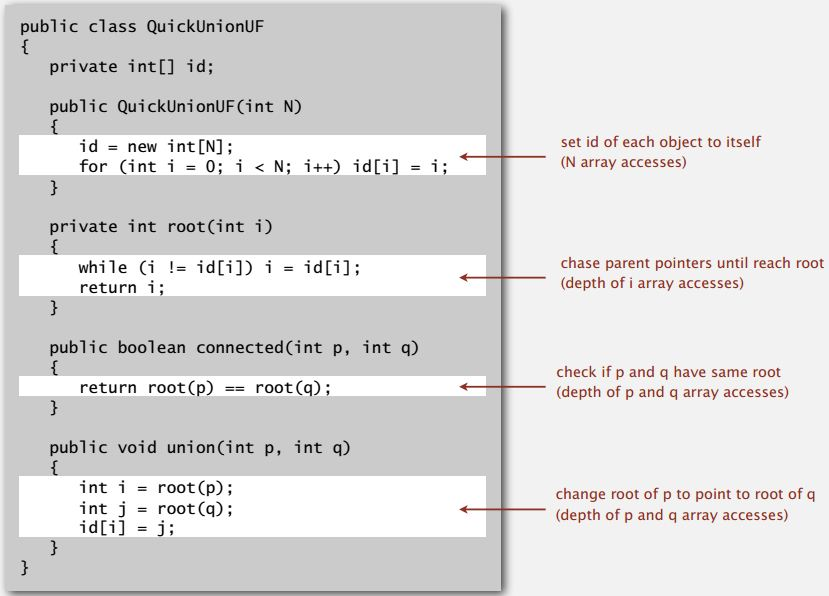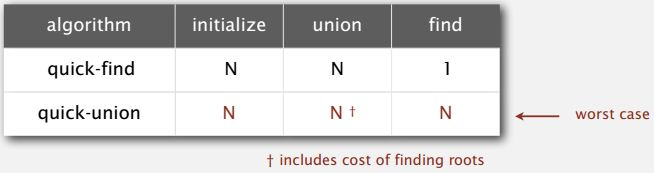### Introduction

We found Quick-find is too slow for huge problems. So, how are we going to do better? Our first attempt is an alternative called, Quick-union.

### Data structure

Quick-union is also called lazy approach to algorithm design where we try to avoid doing work until we have to. It uses the same data structure or array ID with size N but now it has a different interpretation. We are going to think of that array as representing a set of trees that's called a forest as depicted in Figure 2. So, each entry in the array is going to contain a reference to its parent in the tree. For example, 3's parent is four, 4's parent is nine. So 3's entry is four and 4's entry is nine in the array. Now each entry in the array has associated with it a root. That's the root of its tree.Figure 1: Array ID for Quick-unionFigure 2: trees interpretation

In general, the data structure are:

• Integer array id[] of size N
• Interpretation: id[i] is parent of i
• Root of i is id[id[id[...id[i]...]]]

Find operation: check if p and q have the same root. For example, find(3,5)Union operation: to merge components containing p and q, set the id of p's root to the id of q's root. For example, union(3, 5)### Quick-union: Java implementation### Quick-union efficiency (it is also too slow)

Cost model: Number of array accesses (for read and write)Quick-union defect:

• Trees can get very tall
• Find too expensive (could be N array accesses)

• N/A